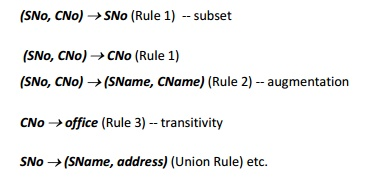Home | | Database Management Systems | Functional Dependencies Definition

# Functional Dependencies Definition

Functional dependency (FD) is a constraint between two sets of attributes from the database.

Functional Dependencies Definition

Functional dependency (FD) is a constraint between two sets of attributes from the database.

o A functional dependency is a property of the semantics or meaning of the attributes. In every relation

R(A1,  A2,…,  An)  there->A1,isA2,a FD…, calledAnFormallythePKthe  FD

o If X and Y are two sets of attributes, that are subsets of T

For any two tuples t1 and t2 in r , if t1[X]=t2[X], we must also have t1[Y]=t2[Y].

Notation: o If the values of Y are determined by the values of X, then it is denoted by X -> Y

o Given the value of one attribute, we can determine the value of another attribute

X f.d. Y or X -> y

Example: Consider the following,

Student Number -> Address, Faculty Number -> Department,

Department Code -> Head of Dept

Functional  dependencies  allow  us  to  expresskeys.  co

Consider the schema:

Loan-info-schema = (customer-name, loan-number,

branch-name, amount).

We expect this set of functional dependencies to hold:

loan-number  amount

loan-number  branch-name

but would not expect the following to hold:

loan-number  customer-name

Use of Functional Dependencies

We use functional dependencies to:

o test relations to see if they are legal under a given set of functional dependencies.

o If a relation r is legal under a set F of functional dependencies, we say that r satisfies F.

o specify constraints on the set of legal relations

o We say that F holds on R if all legal relations on R satisfy the set of functional dependencies F.

Note: A specific instance of a relation schema may satisfy a functional dependency even if the  functional dependency does not hold on all legal instances.

o For example, a specific instance of Loan-schema may, by chance, satisfy loan-number  customer- name.

Example

EmployeeNote: Name is functionally dependent on SSN because from their SSN. Name does not determine SSN, because more than one employee can have the same name.

Keys

Ø        Whereas a key is a set of attributes that uniquely identifies an entire tuple, a functional dependency allows us to express constraints that uniquely identify the values of certain attributes.

However, a candidate key is always a determinant, but a determinant doesn’t  need  to  be a key.

Axioms

Ø        Before we can determine the closure of the relation, Student, we need a set of rules.

Ø        Developed by Armstrong in 1974, there are six rules (axioms) that all possible functional dependencies may be derived from them.Back to the ExampleBased on the rules provided, the following dependencies can be derived.Properties of FDs

X->Y says redundant X-values always cause the redundancy of Y-values.

FDs are given by DBAs or DB designers.

FDs are enforced/guaranteed by DBMS.

Given an instance r of a relation R, we can only determine that some FD is not satisfied by R, but can not determine if an FD is satisfied by R.

Study Material, Lecturing Notes, Assignment, Reference, Wiki description explanation, brief detail
Database Management Systems : Introduction to DBMS : Functional Dependencies Definition |